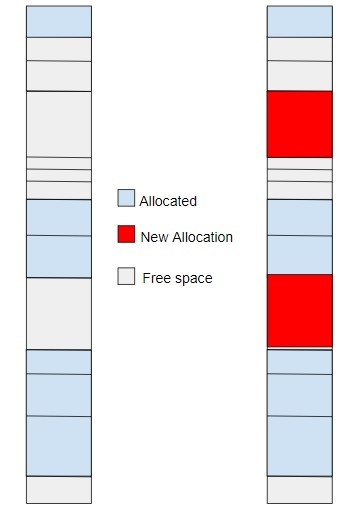# C++ Program for Best Fit algorithm in Memory Management

Given two arrays containing block size and process size; the task is to print the results according to Best Fit algorithm in memory management.

What is Best Fit Algorithm?

Best Fit is a memory management algorithm; it deals with allocating smallest free partition which meets the requirement of the requesting process. In this algorithm we look for the whole memory block and check the smallest and most appropriate block for the process and then look for the immediate near block which can be used to fulfill the adequate process.

So we will take the block size and process size and return the output of the process and which block is to be allocated to a process.## Example

Input: bsize[] = {100, 500, 200, 300, 400}
psize[] = {112, 518, 110, 526}
Output:
Process No. Process Size Block no.
1            112          3
2            518          Not Allocated
3            110          4
4            526         Not Allocated

Approach will be using to solve the above problem −

• Take the input of process ad block size.
• Initially set all the memory blocks as free.
• Take each process and find the minimum block size which can be allotted to a block means the minimum of the entire block which is greater than a process size.
• If found then allot it to the current process else leave that process and check the further process.

## Algorithm

Start
Step 1-> In function void bestfit(int bsize[], int m, int psize[], int n)
Declare int alloc[n]
Call function memset(alloc, -1, sizeof(alloc))
Loop For i=0 and i<n and i++
Declare and Initialize bestIdx = -1
Loop For j=0 and j<m and j++
If bsize[j] >= psize[i] then,
If bestIdx == -1 then,
Set bestIdx = j
Else If bsize[bestIdx] > bsize[j] then,
Set bestIdx = j
If bestIdx != -1 then,
Set alloc[i] = bestIdx
Set bsize[bestIdx] -= psize[i]
Loop For i = 0 and i < n and i++
Print i+1, psize[i]
If alloc[i] != -1
Print alloc[i] + 1
Else
Print "Not Allocated"
Print newline
Step 2->In function int main()
Declare and initialize bsize[] = {100, 500, 200, 300, 400}
Declare and initialize psize[] = {112, 518, 110, 526}
Set m = sizeof(bsize)/sizeof(bsize)
Set n = sizeof(psize)/sizeof(psize)
Call function bestfit(bsize, m, psize, n)
Stop

## Example

#include <iostream>
#include <memory>
using namespace std;
// To allocate the memory to blocks as per Best fit
// algorithm
void bestfit(int bsize[], int m, int psize[], int n) {
// To store block id of the block allocated to a
// process
int alloc[n];
// Initially no block is assigned to any process
memset(alloc, -1, sizeof(alloc));
// pick each process and find suitable blocks
// according to its size ad assign to it
for (int i=0; i<n; i++) {
// Find the best fit block for current process
int bestIdx = -1;
for (int j=0; j<m; j++) {
if (bsize[j] >= psize[i]) {
if (bestIdx == -1)
bestIdx = j;
else if (bsize[bestIdx] > bsize[j])
bestIdx = j;
}
}
// If we could find a block for current process
if (bestIdx != -1) {
// allocate block j to p[i] process
alloc[i] = bestIdx;
// Reduce available memory in this block.
bsize[bestIdx] -= psize[i];
}
}
cout << "\nProcess No.\tProcess Size\tBlock no.\n";
for (int i = 0; i < n; i++) {
cout << " " << i+1 << "\t\t\t\t" << psize[i] << "\t\t\t\t";
if (alloc[i] != -1)
cout << alloc[i] + 1;
else
cout << "Not Allocated";
cout << endl;
}
}
// Driver code
int main() {
int bsize[] = {100, 500, 200, 300, 400};
int psize[] = {112, 518, 110, 526};
int m = sizeof(bsize)/sizeof(bsize);
int n = sizeof(psize)/sizeof(psize);
bestfit(bsize, m, psize, n);
return 0 ;
}

## Output

Process No. Process Size    Block no.
1              112             3
2              518          Not Allocated
3              110             4
4              526          Not Allocated